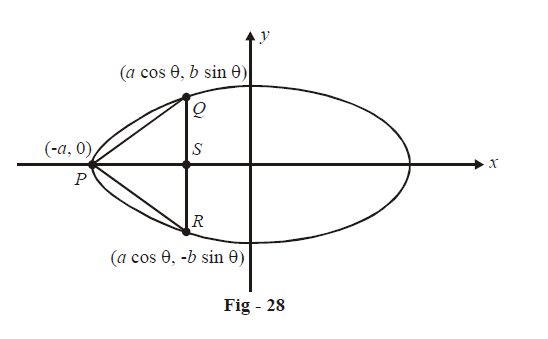# Examples on Maxima and Minima Set 4

Go back to  'Applications of Derivatives'

## Maxima and minima examples

Example - 27

Find the area of the greatest isosceles triangle that can be inscribed in a given ellipse having its vertex coincident with one end of the major axis.

Solution: Assuming the equation of the ellipse to be \begin{align}\frac{{{x^2}}}{{{a^2}}} + \frac{{{y^2}}}{{{b^2}}} = 1,\end{align}  let one vertex of the isosceles triangle be coincident with $$\left( -a,\text{ }0 \right)$$. The other two vertices are variable (though related to each other as mirror reflections).The area of  $$\Delta PQR$$ is

\begin{align}A &= \frac{1}{2} \times QR \times PS\\\\&= \frac{1}{2} \times 2b\sin \theta \times \left( {a + a\cos \theta } \right)\\\\&= ab\sin \theta \left( {1 + \cos \theta } \right)\end{align}

For maximum area,

\begin{align}\frac{{dA}}{{d\theta }}&= 0\,\,{\rm{and }}\frac{{{d^2}A}}{{d{\theta ^2}}} < 0\\\\ \Rightarrow \qquad \frac{{dA}}{{d\theta }}&= ab\left\{ {\cos \theta \left( {1 + \cos \theta } \right) - \sin \theta \cdot \sin \theta } \right\}\\\\&= ab\left\{ {\cos \theta + {{\cos }^2}\theta - {{\sin }^2}\theta } \right\}\\\\&= ab\left\{ {\cos \theta + {{\cos }^2}\theta - 1 + {{\cos }^2}\theta } \right\}\\\\&= ab\left\{ {2{{\cos }^2}\theta + \cos \theta - 1} \right\}\\\\&= ab\left( {2\cos \theta - 1} \right)\left( {\cos \theta + 1} \right)\end{align}

\begin{align}\text{This is 0 when} & \qquad \qquad \cos \theta = \frac{1}{2}\quad\; \Rightarrow \quad \theta = \frac{\pi }{3}\\\text{or} & \qquad \qquad \cos \theta = - 1\quad \Rightarrow \quad \theta = \pi \qquad\text{(obviously a non-valid solution)}\\\end{align}

Verify that \begin{align}{\left. {\frac{{{d^2}A}}{{d{\theta ^2}}}} \right|_{\theta = \pi /3}} < 0\end{align}  so that \begin{align}\theta = \frac{\pi }{3}\end{align}   is a point of local maximum for A.

\begin{align}A_\max &= ab\sin \theta \left( {1 + \cos \theta } \right) |_{\theta = \pi /3}\\ &= ab \times \frac{{\sqrt 3 }}{2} \times \frac{3}{2}\\&= \frac{{3\sqrt 3 ab}}{4}\end{align}

Example – 28

Find the points on the curve $$a{x^2} + {\rm{ }}2bxy + a{y^2} = c, \;0 < \,a\, <\, b\, < c,$$ whose distance from the origin is minimum.

Solution: In some cases, the form of a variable point  on a given curve is obvious from the equation of the curve. For example, we can take a variable point on \begin{align}\frac{{{x^2}}}{{{a^2}}} + \frac{{{y^2}}}{{{b^2}}} = 1\end{align}  as ( a cos $$\theta$$, b sin$$\theta$$) and on    $$y = {x^2}$$  as  $$\left( {t,{t^2}} \right)$$ and so on.

However, if this form is not obvious from the curve, we can take it to be $$({\text{r cos}}\;\theta ,\,\text{r sin}\;\theta )$$ and make this point (which could represent any point on the plane) satisfy the equation of the given curve. We will do this for the current example.

Let (r cos $$\theta$$, r sin $$\theta$$) be a point on the given curve. The co-ordinates therefore must satisfy the equation of the curve:

\begin{align}& a{\left( {rcos{\rm{ }}\theta } \right)^2} + {\rm{ }}2b\left( {rcos{\rm{ }}\theta } \right)\left( {rsin{\rm{ }}\theta } \right){\rm{ }} + a{\left( {rsin{\rm{ }}\theta } \right)^2} = c\\\\ &\Rightarrow \qquad ar{^2} + b{r^2}sin{\rm{ }}2\theta {\rm{ }} = c\\\\ &\Rightarrow \qquad{r^2} = \frac{c}{{a + b\sin 2\theta }}\qquad \qquad \qquad \qquad \rm{(i)} \end{align}

Any point on the curve must satisfy (i). From (i), it is immediately obvious that $${r^2}$$ has a minimum value of \begin{align}\frac{c}{{a + b}}\end{align}  (when $${\rm{sin}}2\theta = 1$$). We were able to obtain the answer without differentiation.

\begin{align}\text{sin 2}\theta &=1\\\\\Rightarrow \qquad 2\theta &= \frac{\pi }{2},\frac{{5\pi }}{2}\\\\\Rightarrow \qquad \,\theta &= \frac{\pi }{4},\frac{{5\pi }}{4} \qquad ( \theta \;\text{must lie in} [0, 2\pi ] \text{so we obtain only two possible values)}\\\\{r_{\min }} &= \sqrt {\frac{c}{{a + b}}} \end{align}

For \begin{align}\theta = \frac{\pi }{4},\end{align}  the required point is $$(\text{r cos}\,\theta, \text{r sin}\,\theta )$$ or \begin{align}\left( {\sqrt {\frac{c}{{2\left( {a + b} \right)}},} \,\,\sqrt {\frac{c}{{2\left( {a + b} \right)}}} } \right)\end{align}

For  \begin{align}\theta = \frac{{5\pi }}{4}\end{align}, the required point is $$(\text{r cos}\;\theta, \text{r sin}\,\theta)$$ or \begin{align}\left( { - \sqrt {\frac{c}{{2\left( {a + b} \right)}}} , - \sqrt {\frac{c}{{2\left( {a + b} \right)}}} } \right)\end{align}